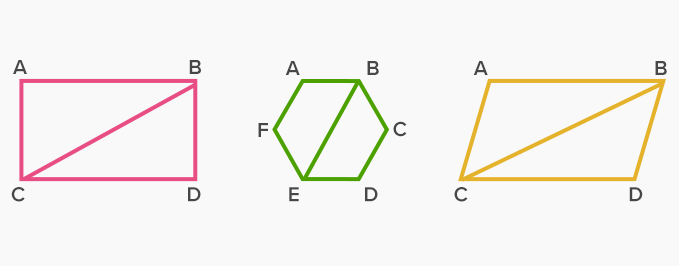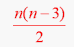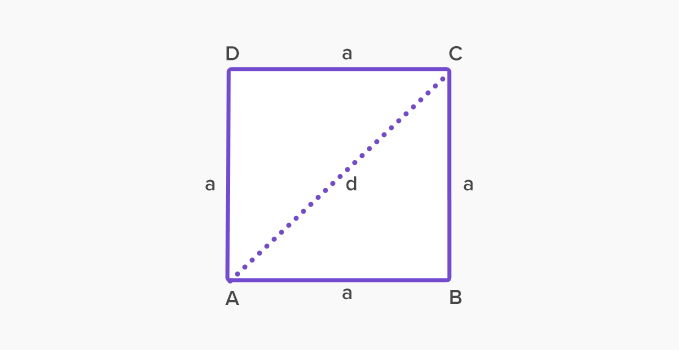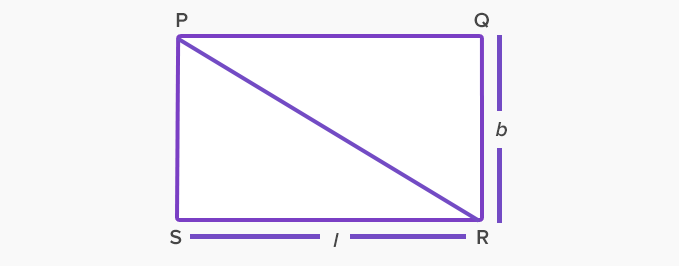# Diagonal - Definition with Examples

The Complete K-5 Math Learning Program Built for Your Child

• 30 Million Kids

Loved by kids and parent worldwide

• 50,000 Schools

Trusted by teachers across schools

• Comprehensive Curriculum

Aligned to Common Core

What is Diagonal?

A diagonal is a straight line connecting the opposite corners of a polygon through its vertex.  To learn about diagonals, we must first know that:

• It (diagonal) is a line segment.

• Polygons are plane figures having at least three sides and angles and usually, it is used to identify figures having five or more sides and angles.

• Vertex is a corner of the shape.

So, diagonal is a line segment connecting two non-adjacent vertices of a polygon. It joins the vertices of a polygon excluding the edges of the figure. The following shapes have a diagonal drawn on them:Some more examples:Here, in the figure of the stop sign, three diagonals are connected by non-consecutive vertices. In the figure the kite, one diagonal is connected by two opposite vertices.

Diagonal formula

Diagonals for polygons of all shapes and sizes can be made and for every shape; there is a formula to determine the number of diagonals.

Number of diagonals in a polygon with n vertices =So, from this formula; we can easily calculate the number of diagonals in a polygon.

The given table shows the number of diagonals in different polygons:

 Shape Names Number of Vertices Number of Diagonals Triangle 3 0 Quadrilateral 4 2 Pentagon 5 5 Hexagon 6 9 Septagon 7 14 Octagon 8 20 Nonagon 9 27 Decagon 10 35

Length of a diagonal

SquareDiagonal of a square = a√2

Therefore, for the above picture, d = a√2

RectangleDiagonal of a rectangle = √l2+b2

 Fun facts about Diagonal The word diagonal comes from Middle French diagonal, Latin diagōnālis, and from Ancient Greek diagṓnios where diá means ‘across’ and gōnía means ‘angle’.

Won Numerous Awards & Honors